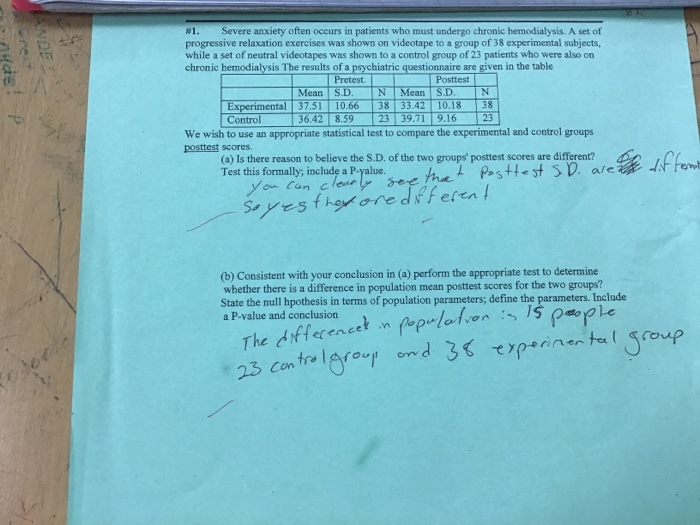# #1. Severe anxiety often occurs in patients who must undergo chronic hemodialysis. A set of progressive...#1. Severe anxiety often occurs in patients who must undergo chronic hemodialysis. A set of progressive relaxation exercises was shown on videotape to a group of 38 experimental subjects, while a set of neutral videotapes was shown to a control group of 23 patients who were also on chronic hemodialysis The results of a psychiatric questionnaire are given in the table Pretest Posttest Mean S.D. N Experimental 37.51 10.66 38 33.42 10.18 38 36.42 8.59 23 39.71 9.16 23 Mean Control We wish to use an appropriate statistical test to compare the experimental and control groups posttest scores. (a) Is there reason to believe the S.D, of the two groups' posttest scores are different? Test this formally, include a P-yalue ou Can cleae (b) Consistent with your conclusion in (a) perform the appropriate test to determine whether there is a difference in population mean posttest scores for the two groups? State the null hpothesis in terms of population parameters; define the parameters. Include a P-value and conclusion Le 23 con trolarou

Solution

Let X = Post-test score of Experimental Group , Y = Post-test score of Experimental Group

Then, X ~ N(µ1, σ12) and Y ~ N(µ2, σ22)

Part (a)

To test equality of population standard deviations.

Hypotheses:

Null H0: σ12 = σ22   Vs Alternative HA: σ12 ≠ σ22

Test statistic:

F= s12/s22where s1and s2 are standard deviations based on n1 observations on X and n2 observations on Y.

= 1.2351

[Note: F is defined as above when s12 > s22. If s22 > s12, F is defined as F = s22/s12]

Calculations

 n1 38 n2 23 s1 10.18 s2 9.16 Fcal 1.235107 Assuming, α = 0.05 Fcrit 1.949457 n1 - 1 37 n2 - 1 22 p-value 0.304313

Distribution, Critical Value and p-value

Under H0, F~ Fn1 – 1, n2 – 1 or Fn2 – 1, n1 – 1 depending on whether s12 > s22 or s22 > s12

Critical value = upper α% point of Fn1 – 1, n2 – 1 or Fn2 – 1, n1 – 1 depending on whether s12 > s22 or s22 > s12

p-value = P(Fn1 – 1, n2 – 1 > F(cal)) or P(Fn2 – 1, n1 – 1 > F(cal)), depending on whether s12 > s22 or s22 > s12

Fcrit and and p-value are found to be as shown in the above table [using Excel Function: Statistical   FINV and FDIST respectively]

Decision:

Since p-value > α. H0 is accepted.

Conclusion:

There is not sufficient evidence to suggest that the population variances and hence the population standard deviations are different for the two groups. Answer

Part (b)

To test equality of population means where σ12 = σ22 = σ2, say but σ2 is unknown [vide Answer 1].

Hypotheses:

Null: H0: µ1 = µ2 Vs Alternative: HA: µ1 µ2

Test Statistic:

t = (Xbar - Ybar)/[s√{(1/n1) + (1/n2)}] where

s2 = {(n1 – 1)s12 + (n2 – 1)s22}/(n1 + n2 – 2);

Xbar and Ybar are sample averages and s1,s2 are sample standard deviations based on n1 observations on X and n2 observations on Y respectively.

= 2.427

Calculations

Summary of Excel calculations is given below:

 n1 38 n2 23 Xbar 33.42 Ybar 39.71 s1 10.18 s2 9.16 s^2 96.27664 s 9.812066 tcal - 2.426504 Assuming α = 0.05 tcrit 2.000995 p-value 0.018319

Distribution, Significance Level, α Critical Value and p-value:

Under H0, t ~ tn1 + n2 - 2. Hence, for level of significance α%, Critical Value = upper (α/2)% point of tn1 + n2 - 2 and p-value = P(tn1 + n2 - 2 > | tcal |) = 2P(tn1 + n2 - 2 > tcal) if tcal > 0 and 2P(tn1 + n2 - 2 < tcal) if tcal < 0

Using Excel Function: Statistical TINV and TDIST, these are found to be as shown in the above table.

Decision:

Since | tcal | > tcrit, or equivalently since p-value < α, H0 is rejected.

Conclusion:

There is sufficient evidence to suggest that the two groups differ with respect to the means. i.e., we conclude that the population means of post-test scores differ from group to group. Answer

DONE